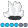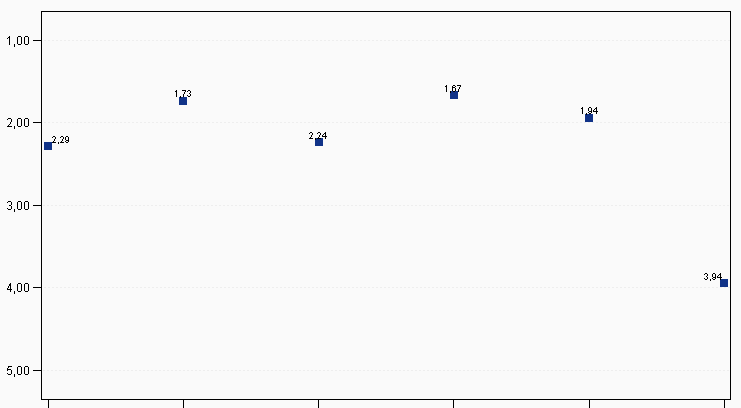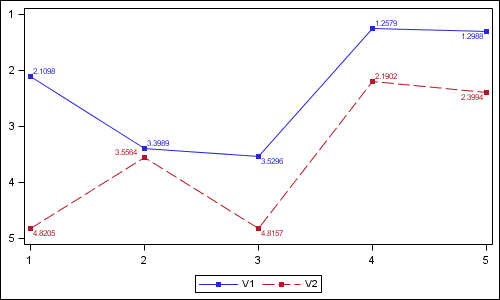Eva
Quartz | Level 8

## gplot problem: values don't connect

Dear all,

My values don't connect in a gplot although I write symbol statements. Does anybody know why this might happen? Here is my code (it shoul produce one line):

symbol1 interpol = join pointlabel height=0.75 line=1 color=CX113388 value=cube;

legend1 label=none position=(bottom center outside);

proc gplot data=mydata;

format num_var1 commax10.2;

plot num_var1 * char_var1 = num_var2 / autovref lautovref = 2 cautovref = CXEEEEEE

vzero

vaxis = axis1 haxis = axis2 vreverse

legend = legend1;

axis1 label=("My label" angle=90) minor=none order=(1 to 5 by 1) offset=(1cm);

axis2 label=none;

run;

quit;

num_var1 and num_var2 are numeric

char_var1 is character

Everything is done from the symbol statement except the interpol=join.(I removed the labels, legend and values on the screenshot as it is company data)

Best wishes

Eva

9 REPLIES 9

## Re: gplot problem: values don't connect

Hi,

One way to control your SAS plots is in 'goption'. For example using SAS example ( it produce a graph like yours):

/* Put this line above your Graph, I put it here. This line is not in SAS example*/

goptions reset=global device=activex ypixels=600 xpixels=800;

data jobs;
length eng \$5;
input eng dollars num;
datalines;
Civil 27308 73273
Aero  29844 70192
Elec  22920 89382
Mech  32816 19601
Chem  28116 25541
Petro 18444 34833
;
title1 "Member Profile";
title2 "Salaries and Number of Member Engineers";
axis1 label=none
offset=(5,5);
axis2 order=(0 to 40000 by 10000)
label=none;
proc gplot data=jobs;
format dollars dollar9. num comma7.0;
bubble dollars*eng=num / haxis=axis1
vaxis=axis2
vminor=1
bcolor=darkred
blabel
bsize=3;
run;
quit;

Then right click on the graph.Select "Graph Properties" and choose what you want. You can connect dots using regression, lines and ... .

## Re: gplot problem: values don't connect

Try leaving off the "= num_var2" in your plot statement.Eva
Quartz | Level 8

## Re: gplot problem: values don't connect

@Mahommad: I want the value to be connected at start, not by manually selecting activex options.

@Robert: this works. But now I manipulate my data so that I have two distinct values of num_var2 in the data file. I'd like to have 2 lines then. So I have to include the "= num_var". Then the values aren't connected anymore 😞

## Re: gplot problem: values don't connect

Can you post some sample data (without real/proprietary values) that reproduces the problem?Eva
Quartz | Level 8

## Re: gplot problem: values don't connect

Funny thing is that when I leave out "= num_var" I get two lines.

I have nearly the same plot with different data where my plot statement is

plot num_var1 * num_var2 = num_var3

i.e. are variables are numeric. This is the only difference. And here I get all lines for all the values of num_var3

Do you think the var type is the problem?

## Re: gplot problem: values don't connect

And are you using device=activex, or device=png? (this might make a difference)Eva
Quartz | Level 8

## Re: gplot problem: values don't connect

I still use activex. Haven't found the time to switch to png.Jay54
Meteorite | Level 14

## Re: gplot problem: values don't connect

Don't know if this issue is resolved.  Here is SGPLOT solution:data values;
do x=1 to 5;
V1= 1+3*ranuni(2);
V2= 2+3*ranuni(2);
output;
end;
run;

ods graphics / reset width=5in height=3in imagename='Series';
proc sgplot data=values;
series x=x y=v1 / markers datalabel=v1 markerattrs=(symbol=squarefilled) ;
series x=x y=v2 / markers datalabel=v2 markerattrs=(symbol=squarefilled) ;
xaxis display=(nolabel);
yaxis display=(nolabel) reverse;
run;Eva
Quartz | Level 8

## Re: gplot problem: values don't connect

Dear all,

in the meantime I found the reason for the problem. The reason is not activex. The problem was in my data. I had a fourth variable which had wrong values. After I fixed that, the plot was shown correctly!

Best wishes

Eva

Discussion stats
• 9 replies
• 974 views
• 0 likes
• 4 in conversation